## Example Questions

← Previous 1 3 4 5 6 7 8 9

### Example Question #1 : Percentage

What percentage of a solution is blood if it contains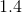ml blood and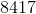ml water?  Round to the nearest thousandth?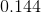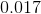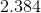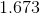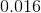Explanation:

First, you must find the total amount of solution.  This is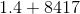, or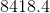.

Now, the percentage of the solution that is blood can be represented: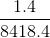, or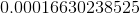This is the same as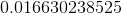%  Rounded, it is%

### Example Question #2 : Percentage

What is the percentage increase of an account that began with a balance of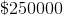and increased to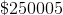?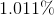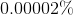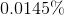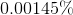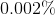Explanation:

Recall that the formula for percentage increase is: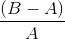, whereis your ending value andis your starting value.

For our numbers, this would be: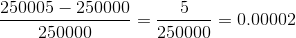Recall that to find a percentage from a decimal, you multiply by: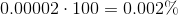### Example Question #3 : Percentage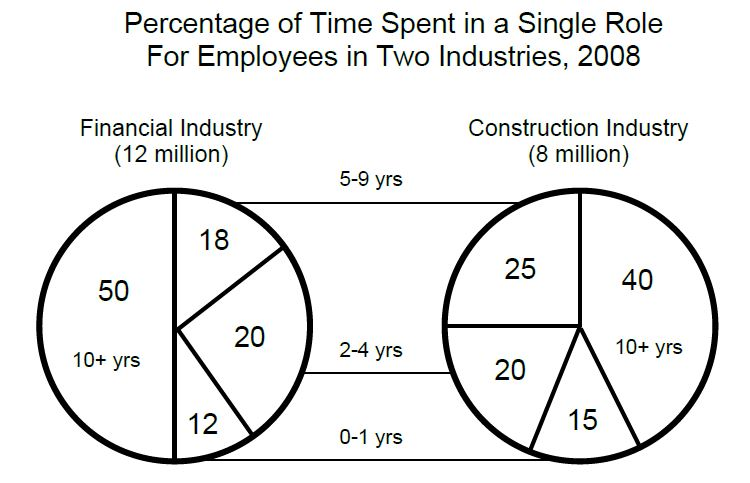If one of the employees across both industries were to be selected at random, what is the probability that the employee will be a construction industry worker who stayed in the same role for 5 years or more?

26%

17%

20%

65%

10%

26%

Explanation:

The first step is to figure out the percentage of construction employees that have stayed in the same role for 5 years or more—this would include both the "5 to 9 year" and "10+ years" ranges. This would be 0.25 + 0.4 = 65% of all construction employees.  To convert to the number of employees, we take the percentage of their total, 0.65 * 8,000,000 = 5,200,000 workers. However, since the probability we are attempting to find is of workers between both industries, we must add the 8 million to the 12 million = 20 million workers total. 5,200,000/20,000,000 = 0.26, or a 26% chance.

### Example Question #4 : Percentage

For every 1000 cookies baked, 34 are oatmeal raisin.

Quantity A: Percent of cookies baked that are oatmeal raisin

Quantity B: 3.4%

Quantity A is greater

The relationship cannot be determined from the information given.

The two quantities are equal.

Quantity B is greater

The two quantities are equal.

Explanation:

Simplify Quantity A by dividing the number of oatmeal raisin cookies by the total number of cookies to find the percentage of oatmeal raisin cookies. Since a percentage is defined as being out of 100, either multiply the resulting decimal by 100 or reduce the fraction until the denominator is 100. You will find that the two quantities are equal.

### Example Question #1 : Percentage

50 students took an exam. There were 4 A's, 9 B's, 15 C's, 8 D's, and the rest of the students failed. What percent of the students failed?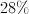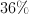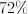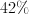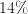Explanation: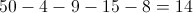students failed.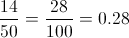which equals 28%.

### Example Question #1 : Percentage

Column A: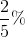Column B: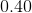The two quantities are equal.

Column A is greater.

Column B is greater.

The relationship cannot be determined from the given information.

Column B is greater.

Explanation:

2/5% = 0.40% = 0.004.  Therefore, Column B is greater.

### Example Question #1 : Percentageispercent ofispercent of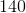Quantity A:Quantity B:The relationship cannot be determined.

Quantity B is greater.

Quantity A is greater.

The two quantities are equal.

Quantity B is greater.

Explanation:

To do this problem, translate each expression into mathematical terms:ispercent of: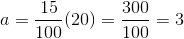ispercent of: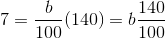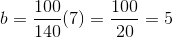Quantity B is greater.

### Example Question #8 : Percentage

Quantity A: 20% ofQuantity B: 30% of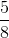Quantity A is greater.

The two quantities are equal.

The relationship cannot be determined.

Quantity B is greater.

Quantity B is greater.

Explanation:

To solve this problem, consider writing the quantities in terms of only fractions:

Quantity A: 20% of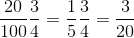Quantity B: 30% of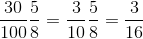Quantity B has the smaller denominator for an equal numerator. There is no need for calculation beyond this, and time on the GRE would be best spent elsewhere.

Quantity B is greater.

### Example Question #2 : Percentage

Robert has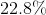of his cereal left.  Choose the answer which best expresses as a decimal, how much of his cereal he has eaten.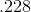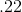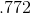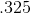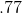Explanation:

The easiest way to convert between percent and decimal is to first convert the percentage into a fraction: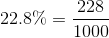Then, from the fraction, you can convert quite easily to a decimal:HOWEVER--in this problem, they are asking you for the portion that makes up the rest of the cereal--so you need to subtract your decimal above from, which yields the correct answer:### Example Question #1 : How To Find Decimal Equivalent To A Percentage

Choose the answer below which best expresses the following as a decimal: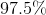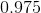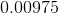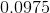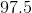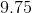Explanation:

When converting percents into decimals, know that the tens and ones digit of the percent will occupy the tenths and hundredths places of the decimal.  Any decimal following the whole percent will follow in sequence (i.e. the first decimal place in a percent corresponds to the thousandth place, and so on).  Therefore, ninety-seven point five percent is equal to: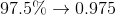← Previous 1 3 4 5 6 7 8 9

Tired of practice problems?

Try live online GRE prep today.